### Home > PC3 > Chapter 8 > Lesson 8.3.4 > Problem8-140

8-140.
1. Determine all values of θ where 0 ≤ θ < 2π. Homework Help ✎

1. csc(θ) =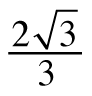2. sec(θ) =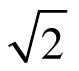3. tan(θ) is undefined

4. cot(θ) = –1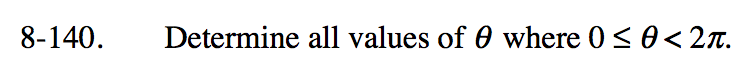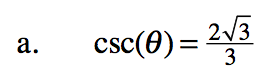$\frac{1}{\sin(\theta)}=\frac{2\sqrt{3}}{3}$

$\sin(\theta)=\frac{3}{2\sqrt{3}}$

$\sin(\theta)=\frac{3}{2\sqrt3}\left(\frac{\sqrt3}{\sqrt3}\right)$

$\sin(\theta)=\frac{\sqrt3}{2}$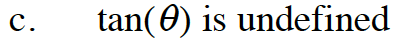$\frac{\sin(\theta)}{\cos(\theta)}=\frac{1}{0}$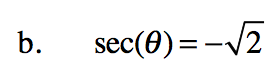$\frac{1}{\cos(\theta)}=-\frac{\sqrt{2}}{1}$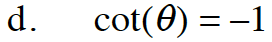$\frac{\cos(\theta)}{\sin(\theta)}=-1$